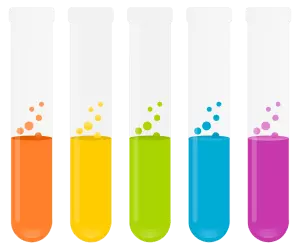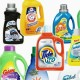# Top 22 Chemistry Interview Questions & Answers

1)      Explain the term Aliquot and Diluent?

• Aliquot: It is a measured sub-volume of the original sample
• Diluent: Material with which sample is diluted

2)      What is molality?

Molality is the number of solutes that are present in 1 kg of a solvent.

3)      What is titration?

Titration is a process to determine the molarity of a base or an acid.  In this process, a reaction is carried out between the known volumes of a solution with a known concentration, against the known volume of a solution with an unknown concentration.

4)      What is a buffer?

A buffer is an aqueous solution which has highly stable pH. It is a blend of a weak acid and its conjugate base or vice versa. On adding a small amount of base or acid to buffer, its pH hardly changes.

5)      How does buffer work?

In buffer when hydrogen ion is added, it will be neutralized by the base in the buffer.  Hydroxide ion will be neutralized by the acid.  On the overall pH of the buffer solution, these neutralization reactions will not show much effect.

While when you select an acid as a buffer solution, try to use an acid that has a pH closed to your desired pH.  This will help your buffer to achieve a nearly equivalent amount of acid and conjugate base, so that it will enable to neutralize as much as H+ and OH -.6)      What is a mole?

Mole is the unit used to define the number of chemical substance present in a substance. It is the amount of substance which consists of the same number of chemical units as there are atoms in exactly 12 gram of pure carbon-12.

7)      How will you calculate how many moles of glucose present in 320 mL of 5.0 M of glucose solution?

First step: Convert the volume from millilitres to litres

• 320  X (1 litre/1000mL) = 0.320 L solution

Second use the formula = M x V

= 5.0 moles glucose/ litre solution X 0.320 L solution

= 1.6 moles of glucose present in 320mL of solution

8)      Explain what is the difference between fractionation and distillation?

Both methods are used to separate the components present in the solution based on the melting points

• Distillation: This technique is used when the boiling point of chemicals are different in the mixtures
• Fractionation: This technique is used when the boiling point of chemicals are close to each other in the mixtures

9)      Mention the formula to calculate pH of a solution?

In order to calculate the pH of a solution you have to use the formula pH= -log [H+] or pH = -log [H3O+]

10)   What is the difference between Molarity and Normality?

Both techniques are used to the amount of chemical present in the solution.  However, they are almost similar but differs in

 Molarity Normality –          Molarity is used to know the total amount of molecules in a 1-liter solution –          Normality is used to know the total number of reactive units in 1 liter of solution –          It is expressed as moles of a compound per litre of solution –          It is expressed in equivalent per litre

11)   What is Valency?

Valency is a property of groups or atoms, equal to the number of atoms of hydrogen that the group or atom could combine with or displace it in forming compounds.

According to Avogadro’s law, at the same temperature and pressure, an equal volume of gases contains the same number of molecules regardless of the chemical nature and physical properties.

Avogadro’s number = 6.023 X 10 (-23)

13)    Explain what makes a molecule into organic molecule?

In a molecule when a hydrogen atom is less than the ratio of the carbon atom, then such molecules are referred to as an organic molecule.

14)   Which metal is used to extract copper from the solution of copper sulfate?

Fe or ferrous is the metal that is used to extract copper from the solution of copper sulphate.

15)   Explain what is the chemical composition of fat in the human body?

The fat found in the human body is mainly composed of

• Glycerides
• Glycerides+Phospholipids
• Glycolipids
• Phosphoinositides
• Tocopherol

16)   What is the monomer of polyethylene?

The monomer of polyethylene is ethylene

17)   What is the formula you will use to calculate how many milliliters of 5.5 M NaOH are required to prepare 400 mL of 1.5M NaOH?

To know the amount or volume of NaOH to prepare 400 mL of 1.5 M NaOH, we use formula

M1 X V1 = M2 X V2

V1 = M2 X V2/ M1

But before that we will convert 400 mL into litre = 0.4 L

5.5 X V1 = 1.5 M x 0.4 L

V1 = 1.5 M X 0.4L/ 5.5

V1= 0.10 L

V1 = 100mL

So, you need 100mL of 5.5 NaOH

18)   Why graphite rod is used in nuclear reactor?

A graphite rod is used in a nuclear reactor allows un-enriched uranium to be used as a nuclear fuel.

19)   Mention how many milliliters is equal to 1 liter and how many microliters is equal to liter?

• 1 millilitre = 0.001 litre
• 1 microliter = 0.0000001 litre

20)   What is oxidation and reduction reaction?

• Oxidation = When there is a loss of hydrogen or electrons OR gain of oxygen is known as Oxidation reaction.
• Reduction = When there is a gain of hydrogen or electron OR loss of oxygen is known as a reduction reaction

Example of oxidation-reduction reaction is observed in the human body when an electron is transferred into the cell and oxidation of glucose take place from which we get the energy.

21)   What does iron ore consist of?

Iron ore is consists of Fe2O3

22)   Explain what is dextro-rotatory and levorotatory?

Levorotation and Dextrorotation are referred to the properties of plane polarized light when light rotates clockwise when it approaches the observer is then known as dextro-rotation, and when the light rotates anti-clockwise then it is referred as levorotation.

A compound which exhibits a dextro-rotation is referred to as dextro-rotatory and which exhibits levorotation is referred to as levorotatory.

31 replies

1.Doris says:

Number 19 isn’t correct. 1ml = 0.001L and so on

•Firdous Hanif sayyed says:

1milliliter =0.0001

•AL W says:

1 millilitre is one 1000th of a litre – therefore 1ml = 0.001L

•updated! Thanks for pointing this out

2.Kishor K says:

How can we determine the boiling points of components in the mixture are far or close to each other?

•Devi says:

How can u determine the rectified spirit

•Yash says:

96% alcohol + 4% water

•basit says:

what is the modern name of element ”sb” ???

3.Amol patil says:

Nice question

4.kundan kumar says:

Practicle questions chemistory

5.ARJUN says:

How to make M/10 solution

•pwoliyan AKSHAI says:

dont know

6.imp question

•Gayake vaibhav says:

Thank you

7.Santhosh chitti says:

Nice qutions

8.Ravina says:

Usefull questions …thnk u for give this questions..

9.Sakthynivedha says:

Pls join ts Grp. Very nice and good

10.Kumar says:

What is range of water

11.Chetan Bagora says:

Very useful questions. thanks

12.Gopabandhu Majhi says:

calculate repulsive force between two electron? when the distance between then is 4.10* -15 (four into ten two the power -15?

13.Murugesan.p says:

good in improview the knowledge questions.

14.Umesh says:

What is Balance shell????

•D. Joys says:

Very useful .what is valance shell

15.cathy says:

•updated! Thanks for pointing this out

16.Sandeepreddy says:

17.Azim Ghanchi says:

Excellent performance for Interview questions once again inter questions basic level

18.Balaabishek says:

Nice

19.Abrham mengstu says:

I am Abrham and I am learning an industrial chemistry in undergraduate level so I want to learn master through fast track. So to get this opportunity there is an interview, please help to answer the interview questions

20.Marmat bhagyashri motilal says:

Give more Question for interview

21.